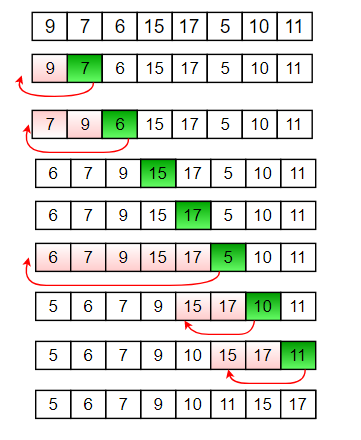# Recursive Insertion Sort

• Difficulty Level : Easy
• Last Updated : 14 Jun, 2022

Insertion sort is a simple sorting algorithm that works the way we sort playing cards in our hands.
Below is an iterative algorithm for insertion sort
Algorithm

```// Sort an arr[] of size n
insertionSort(arr, n)
Loop from i = 1 to n-1.
a) Pick element arr[i] and insert
it into sorted sequence arr[0..i-1] ```

Example:Refer Insertion Sort for more details.
How to implement it recursively?
Recursive Insertion Sort has no performance/implementation advantages, but can be a good question to check one’s understanding of Insertion Sort and recursion.
If we take a closer look at Insertion Sort algorithm, we keep processed elements sorted and insert new elements one by one in the sorted array.
Recursion Idea.

1. Base Case: If array size is 1 or smaller, return.
2. Recursively sort first n-1 elements.
3. Insert last element at its correct position in sorted array.

Below is implementation of above idea.

## C++

 `// Recursive C++ program for insertion sort``#include ``using` `namespace` `std;`` ` `// Recursive function to sort an array using``// insertion sort``void` `insertionSortRecursive(``int` `arr[], ``int` `n)``{``    ``// Base case``    ``if` `(n <= 1)``        ``return``;`` ` `    ``// Sort first n-1 elements``    ``insertionSortRecursive( arr, n-1 );`` ` `    ``// Insert last element at its correct position``    ``// in sorted array.``    ``int` `last = arr[n-1];``    ``int` `j = n-2;`` ` `    ``/* Move elements of arr[0..i-1], that are``    ``greater than key, to one position ahead``    ``of their current position */``    ``while` `(j >= 0 && arr[j] > last)``    ``{``        ``arr[j+1] = arr[j];``        ``j--;``    ``}``    ``arr[j+1] = last;``}`` ` `// A utility function to print an array of size n``void` `printArray(``int` `arr[], ``int` `n)``{``    ``for` `(``int` `i=0; i < n; i++)``        ``cout << arr[i] <<``" "``;``}`` ` `/* Driver program to test insertion sort */``int` `main()``{``    ``int` `arr[] = {12, 11, 13, 5, 6};``    ``int` `n = ``sizeof``(arr)/``sizeof``(arr);`` ` `    ``insertionSortRecursive(arr, n);``    ``printArray(arr, n);`` ` `    ``return` `0;``}`

## C

 `#include `` ` `// Recursive function to sort an array using``// insertion sort``void` `insertionSortRecursive(``int` `arr[], ``int` `n)``{``    ``// Base case``    ``if` `(n <= 1)``        ``return``;`` ` `    ``// Sort first n-1 elements``    ``insertionSortRecursive(arr, n - 1);`` ` `    ``// Insert last element at its correct position``    ``// in sorted array.``    ``int` `last = arr[n - 1];``    ``int` `j = n - 2;`` ` `    ``/* Move elements of arr[0..i-1], that are``    ``greater than key, to one position ahead``    ``of their current position */``    ``while` `(j >= 0 && arr[j] > last) {``        ``arr[j + 1] = arr[j];``        ``j--;``    ``}``    ``arr[j + 1] = last;``}`` ` `// A utility function to print an array of size n``void` `printArray(``int` `arr[], ``int` `size)``{``    ``int` `i;``    ``for` `(i = 0; i < size; i++)``        ``printf``(``"%d "``, arr[i]);``    ``printf``(``"\n"``);``}`` ` `/* Driver program to test insertion sort */``int` `main()``{``    ``int` `arr[] = { 12, 11, 13, 5, 6 };``    ``int` `n = ``sizeof``(arr) / ``sizeof``(arr);`` ` `    ``insertionSortRecursive(arr, n);``    ``printArray(arr, n);`` ` `    ``return` `0;``}`` ` `// This code is contributed by pariveshsrivastava1093`

## Java

 `// Recursive Java program for insertion sort`` ` `import` `java.util.Arrays;`` ` `public` `class` `GFG ``{``    ``// Recursive function to sort an array using``    ``// insertion sort``    ``static` `void` `insertionSortRecursive(``int` `arr[], ``int` `n)``    ``{``        ``// Base case``        ``if` `(n <= ``1``)``            ``return``;``      ` `        ``// Sort first n-1 elements``        ``insertionSortRecursive( arr, n-``1` `);``      ` `        ``// Insert last element at its correct position``        ``// in sorted array.``        ``int` `last = arr[n-``1``];``        ``int` `j = n-``2``;``      ` `        ``/* Move elements of arr[0..i-1], that are``          ``greater than key, to one position ahead``          ``of their current position */``        ``while` `(j >= ``0` `&& arr[j] > last)``        ``{``            ``arr[j+``1``] = arr[j];``            ``j--;``        ``}``        ``arr[j+``1``] = last;``    ``}``     ` `    ``// Driver Method``    ``public` `static` `void` `main(String[] args)``    ``{``        ``int` `arr[] = {``12``, ``11``, ``13``, ``5``, ``6``};``      ` `        ``insertionSortRecursive(arr, arr.length);``         ` `        ``System.out.println(Arrays.toString(arr));``    ``}``}`

## Python3

 `# Recursive Python program for insertion sort``# Recursive function to sort an array using insertion sort`` ` `def` `insertionSortRecursive(arr,n):``    ``# base case``    ``if` `n<``=``1``:``        ``return``     ` `    ``# Sort first n-1 elements``    ``insertionSortRecursive(arr,n``-``1``)``    ``'''Insert last element at its correct position``        ``in sorted array.'''``    ``last ``=` `arr[n``-``1``]``    ``j ``=` `n``-``2``     ` `      ``# Move elements of arr[0..i-1], that are``      ``# greater than key, to one position ahead``      ``# of their current position ``    ``while` `(j>``=``0` `and` `arr[j]>last):``        ``arr[j``+``1``] ``=` `arr[j]``        ``j ``=` `j``-``1`` ` `    ``arr[j``+``1``]``=``last``     ` `# A utility function to print an array of size n``def` `printArray(arr,n):``    ``for` `i ``in` `range``(n):``        ``print``(arr[i],end``=``" "``)`` ` `# Driver program to test insertion sort ``arr ``=` `[``12``,``11``,``13``,``5``,``6``]``n ``=` `len``(arr)``insertionSortRecursive(arr, n)``printArray(arr, n)`` ` `# Contributed by Harsh Valecha`

## C#

 `// Recursive C# program ``// for insertion sort``using` `System;`` ` `class` `GFG``{`` ` `    ``// Recursive function to sort ``    ``// an array using insertion sort``    ``static` `void` `insertionSortRecursive(``int` `[]arr, ``                                       ``int` `n)``    ``{``        ``// Base case``        ``if` `(n <= 1)``            ``return``;``     ` `        ``// Sort first n-1 elements``        ``insertionSortRecursive(arr, n - 1);``     ` `        ``// Insert last element at ``        ``// its correct position``        ``// in sorted array.``        ``int` `last = arr[n - 1];``        ``int` `j = n - 2;``     ` `        ``/* Move elements of arr[0..i-1], ``        ``that are greater than key, to ``        ``one position ahead of their``        ``current position */``        ``while` `(j >= 0 && arr[j] > last)``        ``{``            ``arr[j + 1] = arr[j];``            ``j--;``        ``}``        ``arr[j + 1] = last;``    ``}``     ` `    ``//Driver Code``    ``static` `void` `Main()``    ``{``        ``int` `[]arr = {12, 11, 13, 5, 6};``     ` `        ``insertionSortRecursive(arr, arr.Length);``         ` `        ``for``(``int` `i = 0; i < arr.Length; i++)``        ``Console.Write(arr[i] + ``" "``);``    ``}``}`` ` `// This code is contributed by Sam007`

## PHP

 `= 0 && ``\$arr``[``\$j``] > ``\$last``)``        ``{``            ``\$arr``[``\$j` `+ 1] = ``\$arr``[``\$j``];``            ``\$j``--;``        ``}``        ``\$arr``[``\$j` `+ 1] = ``\$last``;``    ``}``     ` `    ``// A utility function to ``    ``// print an array of size n``    ``function` `printArray(&``\$arr``, ``\$n``)``    ``{``        ``for` `(``\$i` `= 0; ``\$i` `< ``\$n``; ``\$i``++)``            ``echo` `\$arr``[``\$i``].``" "``;``    ``}`` ` `// Driver Code``\$arr` `= ``array``(12, 11, 13, 5, 6);``\$n` `= sizeof(``\$arr``);`` ` `insertionSortRecursive(``\$arr``, ``\$n``);``printArray(``\$arr``, ``\$n``);`` ` `// This code is contributed by ChitraNayal.``?>`

## Javascript

 ``

Output :

`5 6 11 12 13 `

### Check out DSA Self Paced Course

This article is contributed by Sahil Chhabra (akku). If you like GeeksforGeeks and would like to contribute, you can also write an article using write.geeksforgeeks.org or mail your article to review-team@geeksforgeeks.org. See your article appearing on the GeeksforGeeks main page and help other Geeks.